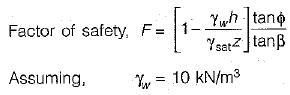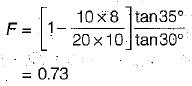Courses

# Test: Stability of Earth Slopes

## 10 Questions MCQ Test GATE Civil Engineering (CE) 2022 Mock Test Series | Test: Stability of Earth Slopes

Description
This mock test of Test: Stability of Earth Slopes for Civil Engineering (CE) helps you for every Civil Engineering (CE) entrance exam. This contains 10 Multiple Choice Questions for Civil Engineering (CE) Test: Stability of Earth Slopes (mcq) to study with solutions a complete question bank. The solved questions answers in this Test: Stability of Earth Slopes quiz give you a good mix of easy questions and tough questions. Civil Engineering (CE) students definitely take this Test: Stability of Earth Slopes exercise for a better result in the exam. You can find other Test: Stability of Earth Slopes extra questions, long questions & short questions for Civil Engineering (CE) on EduRev as well by searching above.
QUESTION: 1

### A cohesionless soil having an angle of shearing resistant of ϕ, is standing at a slope angle of i. The factor of safety of the slope is:

Solution:

For an infinite slope in cohesionless soil.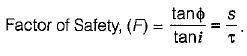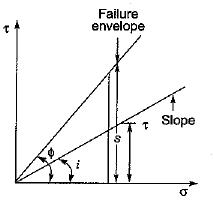For ϕ > i F > 1 it means that the slope remains stable as long as angle of slope is less than angle of shearing resistance.

QUESTION: 2

### A finite slope of cohesion less soil is safe so long as

Solution:

The factor of safety of slope against shear failure is given by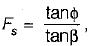from which
if ϕ = β; slope is just stable
ϕ < β; slope is unstable
ϕ > β; slope is stable

QUESTION: 3

### In the friction circle method the radius of the friction circle is given by

Solution:QUESTION: 4

Taylor's stability number is equal to
where c = unit cohesion, Fc = factor of safety with respect to cohesion, γ = unit weight of soil, Hc = critical height, H = actual height

Solution:

Taylor’s stability No.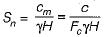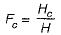​∴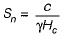QUESTION: 5

If the unit weight of soil is 20 kN/m3 cohesion is 15 kN/m2, factor of safety is 1.5 and the stability number is 0.05, the safe maximum height of the slope is

Solution:

Stability number,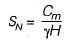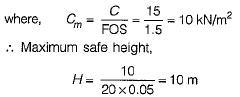QUESTION: 6

An infinite soil slope with an inclination of 35° is subjected to seepage parallel to its surface. The soil has c' = 100 kN/m2 and ϕ = 30°. Using the concept of mobilized cohesion and friction, at a factor of safety of 1.5 with respect to shear strength, the mobilized friction angle is

Solution:

The factor of safety with respect to shear strength is given by,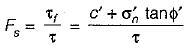The shear strength mobilised, thus may be written as,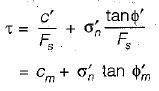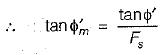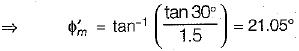QUESTION: 7

List-I given below gives the possible types of failure for a finite soil slope and List-II gives the reasons for these different types of failure. Match the items in List-I with the items in List-II and select the correct answer from the codes given below the lists:
List-I
A. Base failure
B. Face failure
C. Toe failure
List-II
1. Soils above and below the toe have same strength
2. Soil above the toe is comparatively weaker
3. Soil above the toe is comparatively stronger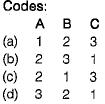Solution:

Face failure or slope failure can occur when the slope angle β is very high and the soil close to the toe is quite strong or the soil in the upper part of slope is relatively weak.
Base failure can occur when the soil below the toe is relatively weak and soft and the slope is flat. Toe failure occurs in steep slopes when the soil mass above the base and below the base is homogeneous.

QUESTION: 8

Taylor’s stability number curves are used for the analysis of stability of slopes. The angle of shearing resistance used in the chart is the:

Solution:

In Taylor's stability chart, the stability number is based on the assumption that factor of safety with respect to friction is unity. Thus effective angle is used in the chart and it is equal to the mobilised angle.

QUESTION: 9

An excavation was made at a slope angle of 54° in homogeneous clay. When the depth of excavation reached 8m, a slip occurred. The slip surface was likely to have passed through a point:

Solution:

For slopes greater than 53°, the critical slip circle invariably passes through the toe. (Toe failure). This is true for any angle of friction (ϕ).
For slopes less than 53° and small values of ϕ < 3°, the critical surface passes below the toe and it is called base failure.

QUESTION: 10

The factor of safety of an infinite soil slope shown in the figure having the properties c = 0, ϕ = 35°, γdry = 16kN/m3 and γsat = 20 kN/m3 is approximately equal to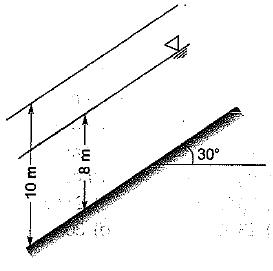Solution: